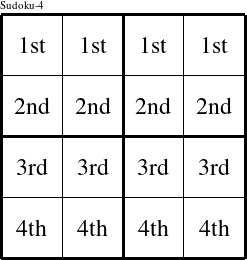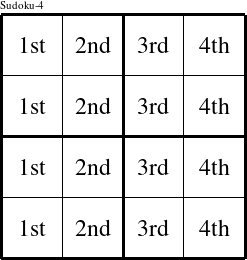#### Printable version

Detailed instructions for working a group puzzle of type 'Suzy'

This is a signature puzzle named 'Suzy'

The 4 elements in each group of this type of puzzle are: S, U, Z, and Y.

Every group in the puzzle must contain exactly this set of elements.

####In a Suzy puzzle, each row is a group numbered as shown in the figure above.

####In a Suzy puzzle, each column is a group numbered as shown in the figure above.

####In a Suzy puzzle, each 2x2 square is a group numbered as shown in the figure above.